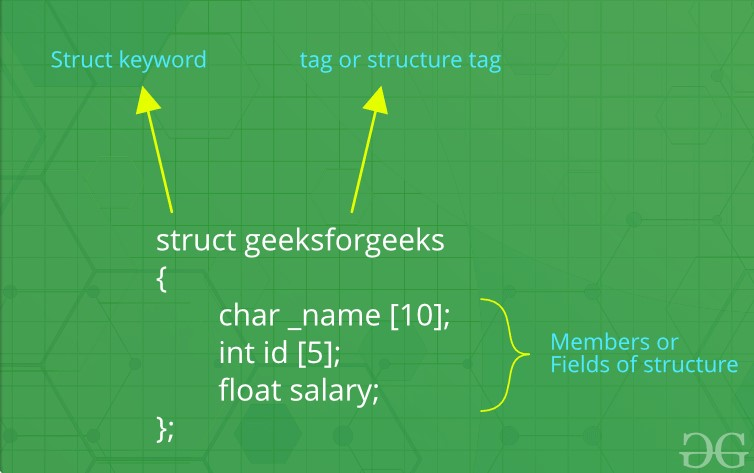Open In App
Related Articles
• Write an Interview Experience
• C++ Programming Language

# Structures in C++

We often come around situations where we need to store a group of data whether of similar data types or non-similar data types. We have seen Arrays in C++ which are used to store set of data of similar data types at contiguous memory locations.
Unlike Arrays, Structures in C++ are user defined data types which are used to store group of items of non-similar data types.

What is a structure?

A structure is a user-defined data type in C/C++. A structure creates a data type that can be used to group items of possibly different types into a single type.Structures in C++

How to create a structure?

The ‘struct’ keyword is used to create a structure. The general syntax to create a structure is as shown below:

```struct structureName{
member1;
member2;
member3;
.
.
.
memberN;
};```

Structures in C++ can contain two types of members:

• Data Member: These members are normal C++ variables. We can create a structure with variables of different data types in C++.
• Member Functions: These members are normal C++ functions. Along with variables, we can also include functions inside a structure declaration.

Example:

## C++

 `// Data Members``int` `roll;``int` `age;``int` `marks;``    ` `// Member Functions``void` `printDetails()``{``    ``cout<<``"Roll = "``<

In the above structure, the data members are three integer variables to store roll number, age and marks of any student and the member function is printDetails() which is printing all of the above details of any student.

How to declare structure variables?

A structure variable can either be declared with structure declaration or as a separate declaration like basic types.

## C++

 `// A variable declaration with structure declaration.``struct` `Point``{``   ``int` `x, y;``} p1;  ``// The variable p1 is declared with 'Point'`  `// A variable declaration like basic data types``struct` `Point``{``   ``int` `x, y;``};` `int` `main()``{``   ``struct` `Point p1;  ``// The variable p1 is declared like a normal variable``}`

Note: In C++, the struct keyword is optional before in declaration of a variable. In C, it is mandatory.

How to initialize structure members?
Structure members cannot be initialized with declaration. For example the following C program fails in compilation.
But is considered correct in C++11 and above.

## C++

 `struct` `Point``{``   ``int` `x = 0;  ``// COMPILER ERROR:  cannot initialize members here``   ``int` `y = 0;  ``// COMPILER ERROR:  cannot initialize members here``};`

The reason for above error is simple, when a datatype is declared, no memory is allocated for it. Memory is allocated only when variables are created.

Structure members can be initialized with declaration in C++. For Example the following  C++ program Executes Successfully without throwing any Error.

## C++

 `// In C++ We can Initialize the Variables with Declaration in Structure.` `#include ``using` `namespace` `std;` `struct` `Point {``    ``int` `x = 0; ``// It is Considered as Default Arguments and no Error is Raised``    ``int` `y = 1;``};` `int` `main()``{``    ``struct` `Point p1;` `    ``// Accessing members of point p1``    ``// No value is Initialized then the default value is considered. ie x=0 and y=1;``    ``cout << ``"x = "` `<< p1.x << ``", y = "` `<< p1.y<

``` x=0, y=1
x=0, y=20```

Structure members can be initialized using curly braces ‘{}’. For example, following is a valid initialization.

## C++

 `struct` `Point {``    ``int` `x, y;``};` `int` `main()``{``    ``// A valid initialization. member x gets value 0 and y``    ``// gets value 1.  The order of declaration is followed.``    ``struct` `Point p1 = { 0, 1 };``}`

How to access structure elements?
Structure members are accessed using dot (.) operator.

## C++

 `#include ``using` `namespace` `std;` `struct` `Point {``    ``int` `x, y;``};` `int` `main()``{``    ``struct` `Point p1 = { 0, 1 };` `    ``// Accessing members of point p1``    ``p1.x = 20;``    ``cout << ``"x = "` `<< p1.x << ``", y = "` `<< p1.y;` `    ``return` `0;``}`

Output

`x = 20, y = 1`

What is an array of structures?

Like other primitive data types, we can create an array of structures.

## C++

 `#include ``using` `namespace` `std;` `struct` `Point {``    ``int` `x, y;``};` `int` `main()``{``    ``// Create an array of structures``    ``struct` `Point arr;` `    ``// Access array members``    ``arr.x = 10;``    ``arr.y = 20;` `    ``cout << arr.x << ``" "` `<< arr.y;``    ``return` `0;``}`

Output

`10 20`

What is a structure pointer?
Like primitive types, we can have pointer to a structure. If we have a pointer to structure, members are accessed using arrow ( -> ) operator instead of the dot (.) operator.

## C++

 `#include ``using` `namespace` `std;` `struct` `Point {``    ``int` `x, y;``};` `int` `main()``{``    ``struct` `Point p1 = { 1, 2 };` `    ``// p2 is a pointer to structure p1``    ``struct` `Point* p2 = &p1;` `    ``// Accessing structure members using``    ``// structure pointer``    ``cout << p2->x << ``" "` `<< p2->y;``    ``return` `0;``}`

Output

`1 2`

What is structure member alignment?